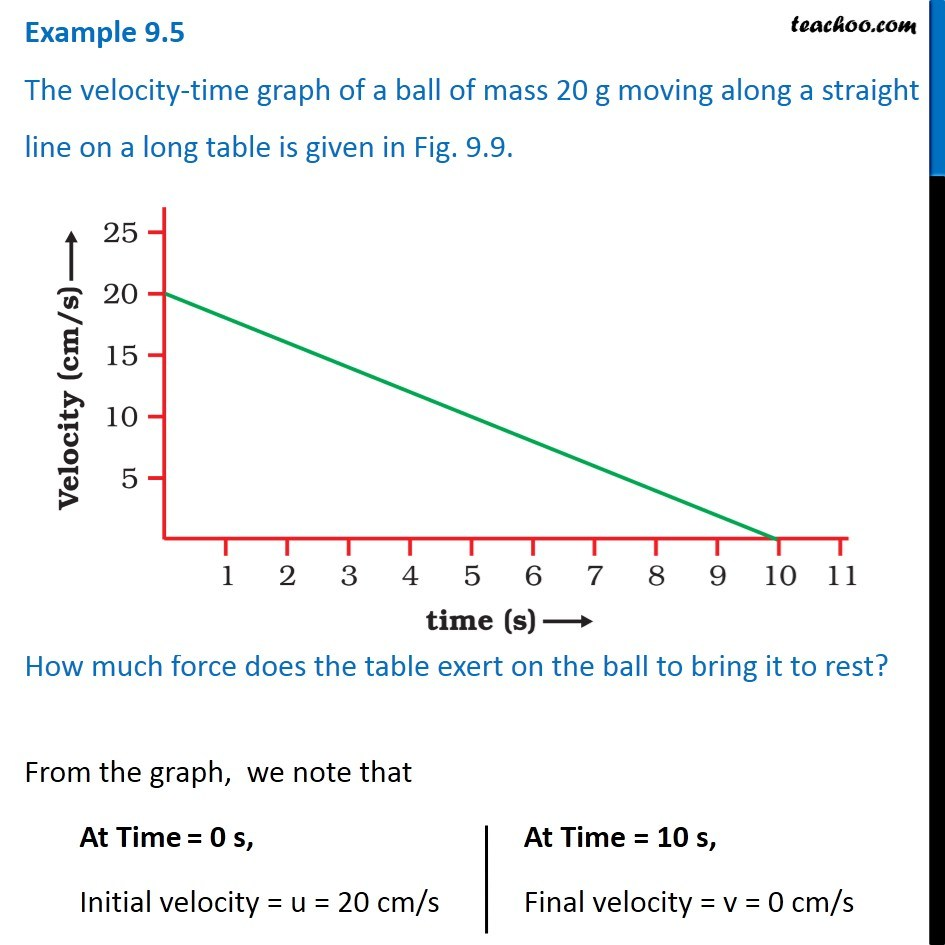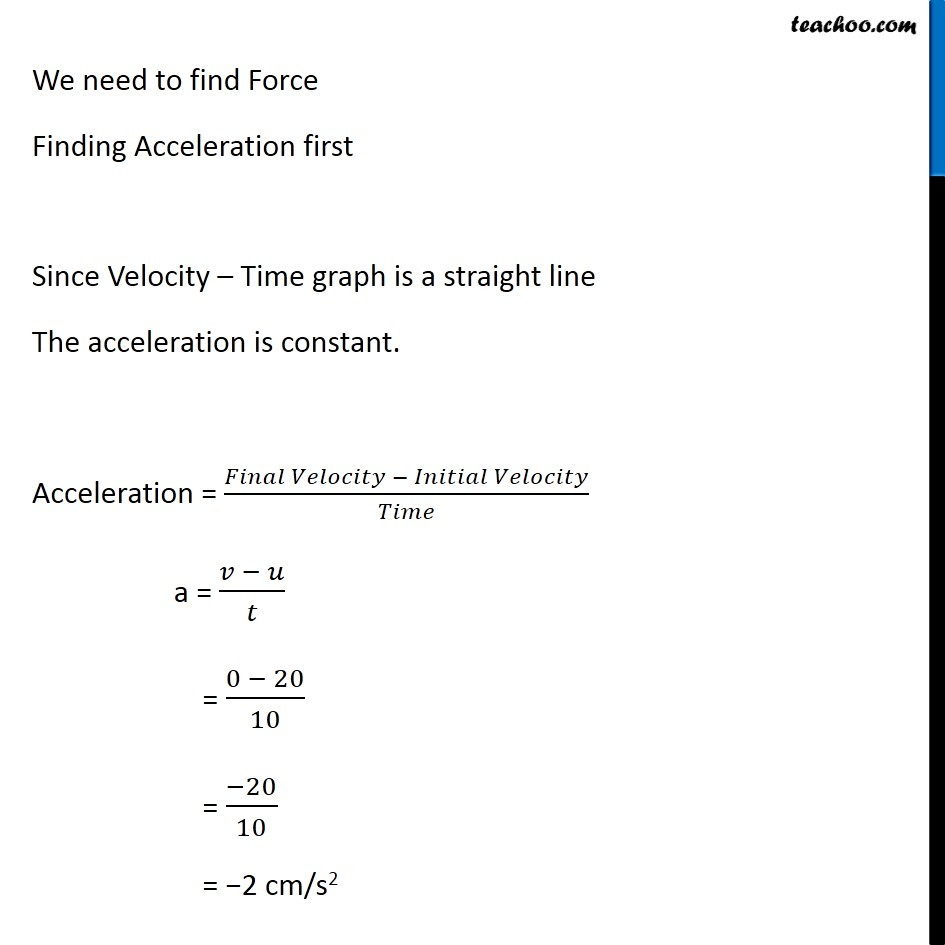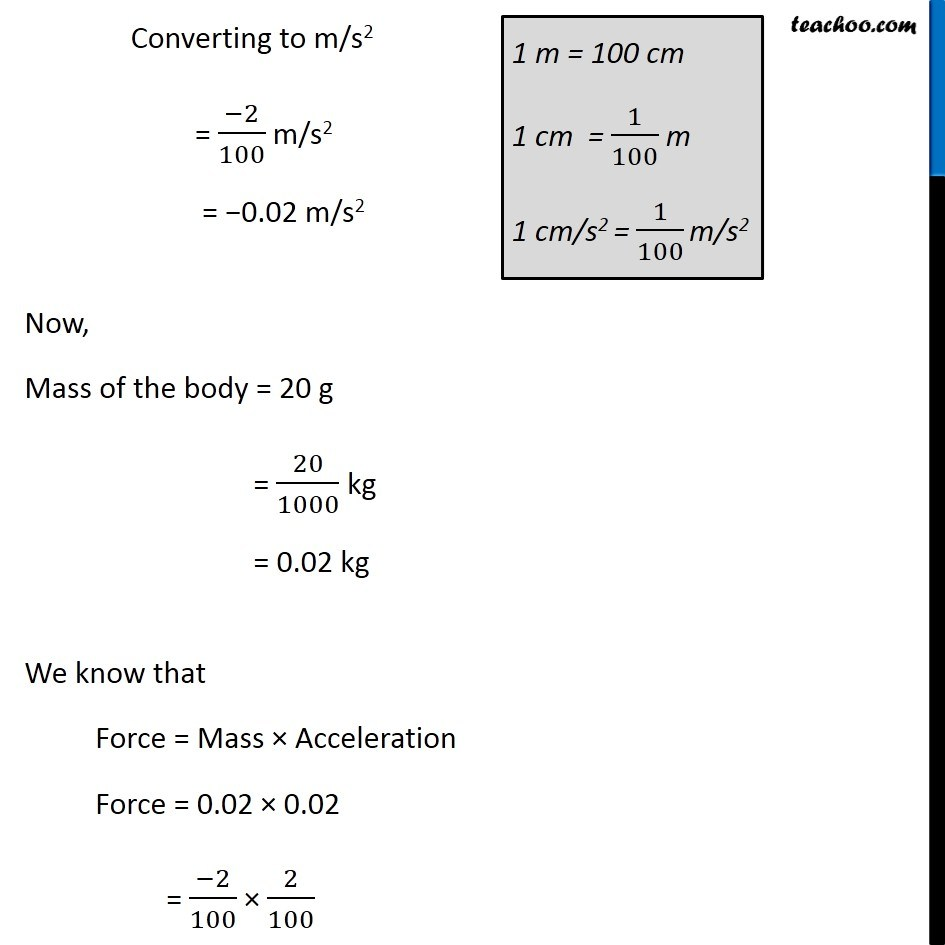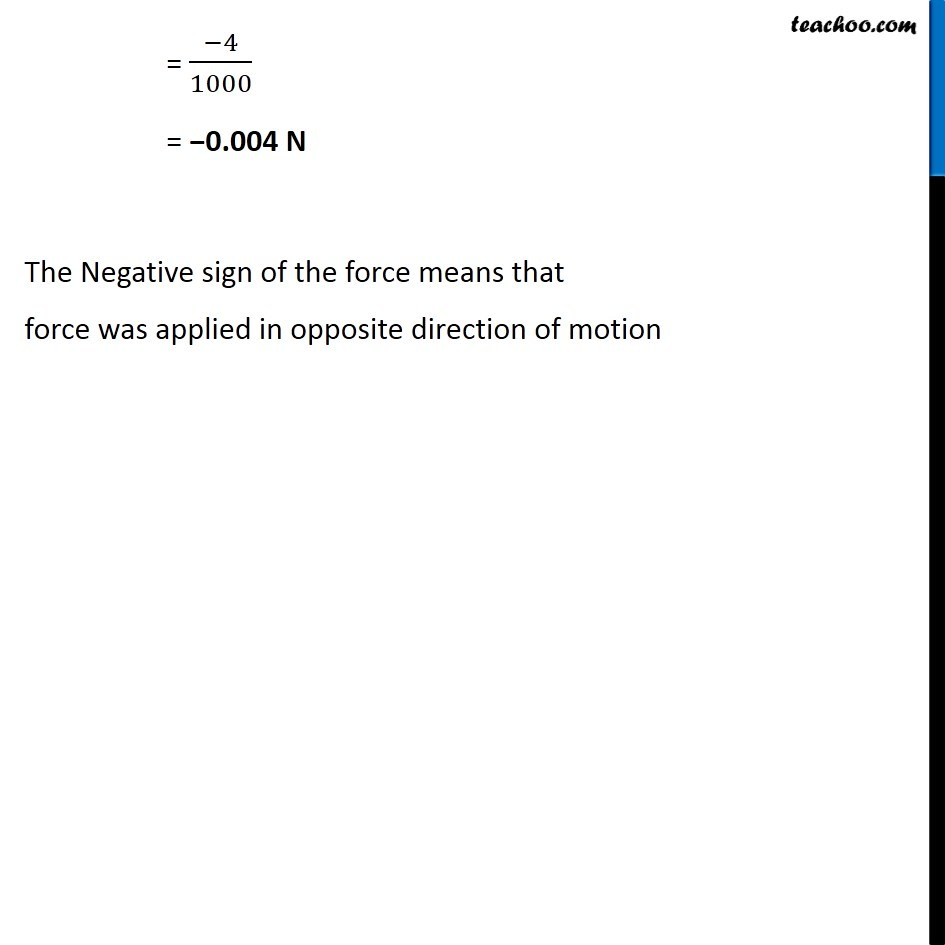Examples from NCERT Book

Class 9
Chapter 9 Class 9 - Force and Laws Of Motion### Transcript

Example 9.5 The velocity-time graph of a ball of mass 20 g moving along a straight line on a long table is given in Fig. 9.9. How much force does the table exert on the ball to bring it to rest? How much force does the table exert on the ball to bring it to rest? From the graph, we note that At Time = 0 s, Initial velocity = u = 20 cm/s At Time = 10 s, Final velocity = v = 0 cm/s We need to find Force Finding Acceleration first Since Velocity – Time graph is a straight line The acceleration is constant. Acceleration = (𝐹𝑖𝑛𝑎𝑙 𝑉𝑒𝑙𝑜𝑐𝑖𝑡𝑦 − 𝐼𝑛𝑖𝑡𝑖𝑎𝑙 𝑉𝑒𝑙𝑜𝑐𝑖𝑡𝑦)/𝑇𝑖𝑚𝑒 a = (𝑣 − 𝑢)/𝑡 = (0 − 20)/10 = (−20)/10 = −2 cm/s2 Converting to m/s2 = (−2)/100 m/s2 = −0.02 m/s2 Now, Mass of the body = 20 g = 20/1000 kg = 0.02 kg We know that Force = Mass × Acceleration Force = 0.02 × 0.02 = (−2)/100 × 2/100 1 m = 100 cm 1 cm = 1/100 m 1 cm/s2 = 1/100 m/s2 = (−4)/1000 = −0.004 N The Negative sign of the force means that force was applied in opposite direction of motion